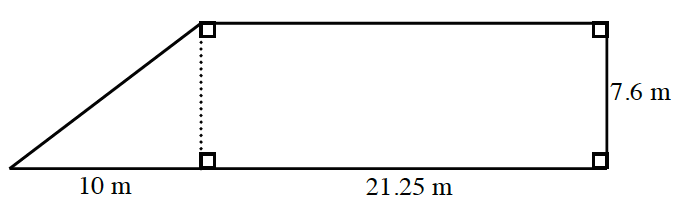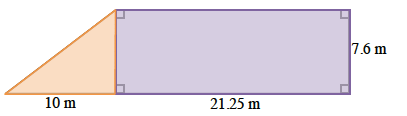Home > CC2MN > Chapter cc18 > Lesson cc18.1.2 > Problem8-23

8-23.

Find the area of the shape at right. Show your steps. Homework Help ✎Start by finding the areas of each separate shape.

$\text{Area of Triangle: } \frac{1}{2}\text{ (base)(height)}$Area of Rectangle: (length)(width)

After you find the area of each separate shape, add the two areas together to find the total area of the shape.

Total Area: $199.5 \text{ m}^2$
Make sure you know how to get this answer.